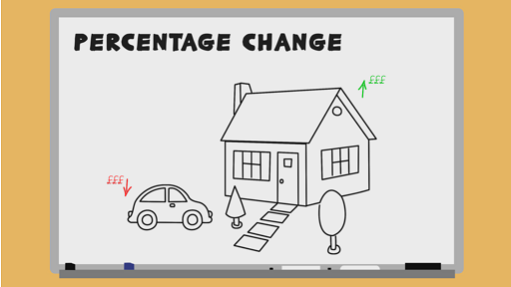Everyday maths 2

Start this free course now. Just create an account and sign in. Enrol and complete the course for a free statement of participation or digital badge if available.

Free course

# 5.2 Percentage change

Watch the video below on how to calculate percentage change, then complete Activity 10.

Download this video clip.Video player: bltl_1_5_2_percentage.mp4Skip transcript

#### Transcript

You will already be familiar with the concept that if you buy a new car, when you come to sell it, its value is likely to have decreased. On the other hand, if you're lucky, when you buy a house and then wish to sell, you may be able to sell it for more than you bought it for.

The percentage difference between the original price and the sale price is called percentage change. This can be useful for working out the percentage profit, or loss, or finding out by what percentage an item has changed in value. It's important to recognise that percentage change can be either positive, if the price has increased, or negative, if the value of the item has gone down.

To calculate the percentage change, we need to use the simple formula: percentage change equals the difference, divided by the original, multiplied by 100. 'Difference' refers to the difference between the two values. That is, the cost before and after. 'Original' means the original value of the item.

Let's use this formula in an example. A train ticket used to cost £24. The price has gone up to £27.60. How do we find the percentage increase? First, you need to find the difference in cost. The difference = £27.60 - £24, which is £3.60.

Next, divide this difference by the original cost, and then multiply by 100. £3.60 divided by £24, x 100, = 15. The cost of the train ticket has increased by 15%.

Now try another example. You bought a car for £4,500 and you managed to sell it for £3,200. What is the percentage decrease? You should give your answer to two decimal places. The difference is £4,500 - £3,200, which = £1,300. Divide this by the original cost of £4,500. 1,300 divided by 4,500, x 100, equals a 28.89% per cent decrease. Now practise using the percentage change formula in the next activity.

End transcript

Interactive feature not available in single page view (see it in standard view).

## Activity 10: Percentage change formula

Practise using the percentage change formula which you learned about in the video above on the three questions below. Where rounding is required, give your answer to two decimal places.

1. Last year, your season ticket for the train cost £1300. This year the cost has risen to £1450. What is the percentage increase?

### Answer

1. Difference: £1450 − £1300 = £150
• a.Original: £1300
• b.Percentage change = £150 ÷ £1300 × 100 = 11.54% increase (rounded to two d.p.)
1. You bought your house 10 years ago for £155,000. You are able to sell your house for £180,000. What is the percentage increase the house has made?

### Answer

1. Difference: £180,000 − £155,000 = £25,000
• a.Original: £155,000
• b.Percentage change = £25,000 ÷ £155,000 × 100 = 16.13% increase (rounded to two d.p.)
1. You purchased your car 3 years ago for £4200. You sell it to a buyer for £3600. What is the percentage decrease of the car?

### Answer

1. Difference: £4200 − £3600 = £600
• a.Original: £4200
• b.Percentage change = £600 ÷ £4200 × 100 = 14.29% decrease (rounded to two d.p.)
FSM_2

### Take your learning further

Making the decision to study can be a big step, which is why you'll want a trusted University. The Open University has 50 years’ experience delivering flexible learning and 170,000 students are studying with us right now. Take a look at all Open University courses.

If you are new to University-level study, we offer two introductory routes to our qualifications. You could either choose to start with an Access module, or a module which allows you to count your previous learning towards an Open University qualification. Read our guide on Where to take your learning next for more information.

Not ready for formal University study? Then browse over 1000 free courses on OpenLearn and sign up to our newsletter to hear about new free courses as they are released.

Every year, thousands of students decide to study with The Open University. With over 120 qualifications, we’ve got the right course for you.

Request an Open University prospectus371